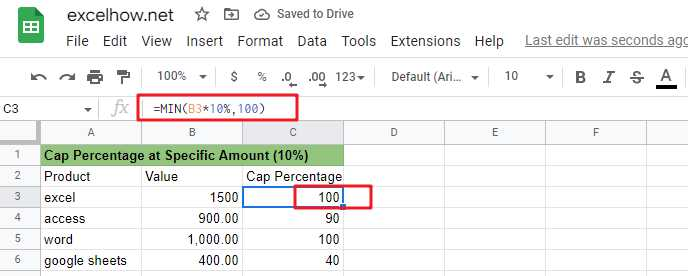# Calculate Cap Percentages to Specific ValueIf you are a newbie on Excel or google Sheets, you may be able to do this task manually. This method works for tasks with a small amount of data, but it is not a wise method for large amounts of data because it will consume a lot of your time.

How can you quickly calculate the cap percentage of a set of amount values? When you calculated the cap percentage of the amount, how do you limit that value to a given value? You can read the following section where we will explain how to do this with formulas in Microsoft Excel or google sheets.

## Calculate the cap percentage on a given amount

You can use the MIN function in Google Sheets or Microsoft Excel Spreadsheet to limit the cap percentage of a given amount to a specific value.

Suppose we have an amount value of 1500 and we need to calculate the upper limit of ten percent of that amount value and return 100 if the calculated cap value is greater than 100, or return that cap value if the calculated cap value is less than 100.

The general formula to complete this task is as follows.

`=MIN(B1*percentage,100)`

By executing this formula, you can always limit the cap percentage value of the amount value to 100.### Explanation of the formula

Let’s look at how this formula works：

Here are the three logics implemented in this formula.

• The MIN formula returns the cap percentage value when the calculated cap percentage value is less than 100.
• The MIN formula returns 100 when the calculated upper percentage value is greater than 100.
• The MIN formula returns 100 when the calculated upper percentage value is equal to 100.

From the above explanation, we can see that the MIN function can return the smallest value between the two values, but also can be used to achieve similar to the IF function can be used to select a specific value of the processing logic. Therefore, sometimes we can also use the MIN function instead of the IF function to complete our task.